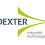# Law of Electromagnetic Induction | cbse24.com

### Electromagnetic Induction:

The phenomenon of production of induced emf(and hence induced current) due to a change of magnetic flux linked with a closed circuit is called electromagnetic induction.

The phenomenon of electromagnetic induction was discovered and understood on the basis of the following experiments performed by Faraday and Henery.

### Experiment:

Induced emf with a stationary coil and moving magnetInduced emf with a moving coil magnet and stationary coil

• When the N-pole of a strong bar magnet is moved towards the coil, the galvanometer shows a deflection , ay to the right of the zero marks in fig1.
• When the N-pole of the bar magnet is moved away from the coil, the galvanometer shows a deflection in the direction(fig2).
• When the magnet is held stationary anywhere near or inside the coil, the galvanometer does not show any deflection

### Law of Electromagnetic Induction:

There are two types of laws which governs the phenomenon of electromagnetic induction

1. Faraday's laws which give us the magnitude of induced emf
2. Lenz's law which gives us the direction of induced emf

### A. Faraday's law of electromagnetic induction:

These can be stated as follows:

|e|=dϕdt

### B.Lenz's Law:

This law states that the direction of induced current is such that it opposes the cause which produces it i.e it opposes the change in magnetic flux.

### The mathematical form of the laws of electromagnetic induction:

According to the Faraday's flux rule,

The magnitude of induced emf=Rate of change of magnetic flux

|e|=dϕdt

Taking into account Lenz's rule for the direction of induced emf,Faraday's law takes the form

e=dϕdt

The negative sign indicates that the direction of induced emf is such that it opposes the change in magnetic flux.

If the flux changes from ϕ1 to ϕ2 in time t then the average induced end will be.

e=Nϕ2ϕ1t

If Φ is in webers and t in seconds, then e will be in volts

1.2.